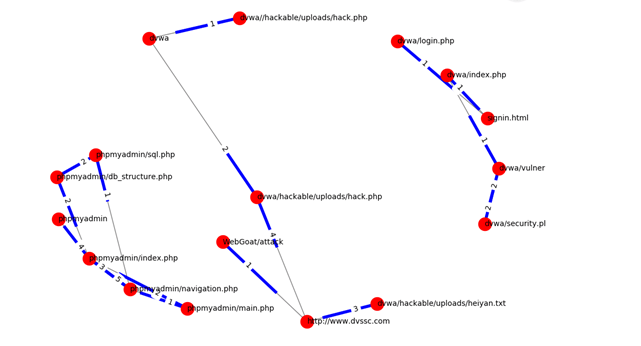### Spark数据分析之有向图

graphframe是将spark中的graph算法统一到DataFrame接口的Graph操作接口，支持多种语言，可以供python使用。

### 安装配置环境

grameframe的源码，然后把中间的python目录放到我们的python三方库路径就可以

spark依赖：spark解析需要一个包graphframes:0.2.0-spark1.6-s_2.10

``````
os.environ["PYSPARK_SUBMIT_ARGS"] = (
"--packages graphframes:graphframes:0.2.0-spark1.6-s_2.10 pyspark-shell"
)
``````

### 清楚自己可以调用那些接口

graphframe里面我们使用方式有：

1.先生成graph对象：

``````
G = graphframe.GraphFrame(verice,edges)
``````

2.可以调用的方法接口：

outDegree(）所有节点的出度

inDegree()所有节点的入度

bsf()宽度优先搜索，查找节点的最短路径

pageRank()按照其他节点指向它的数目来排序，指向一个节点的越多，这个节点就越重要

vertice打印出顶点

edges打印出边

### 具体使用

g = dataframe.GraphFrame(vertices,edges)

g.inDegree()

g.outDegree()

### 画图

``````
import networkx as nx

graph = nx.DiGraph（）

pos = nx.nx.spring_layout(graph, scale=2, center=(4,5)) # positions for all nodes
# 首先画出节点位置
# nodes
nx.draw_networkx_nodes(graph, pos, node_size=300,node_color='r')
edge_labels = nx.get_edge_attributes(graph,'weight')

# labels标签定义
nx.draw_networkx_labels(graph, pos, font_size=10, font_family='sans-serif', \
horizontalalignment="left")
nx.draw_networkx_edges(graph, pos,alpha=0.5)
nx.draw_networkx_edge_labels(graph,pos,edge_labels = edge_labels,label_pos=0.3)

#nx.graw(graph)
plt.axis('off')
plt.savefig("weighted_graph.png")  # save as png
plt.show()  # display

``````